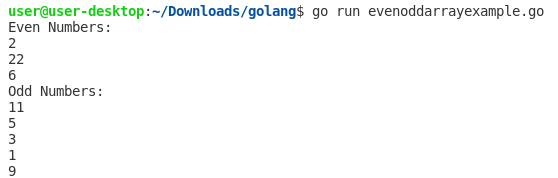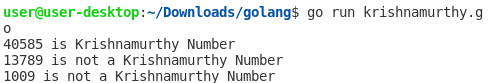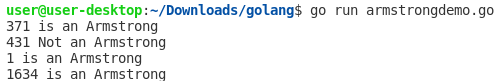## Posts

Showing posts from April, 2021

### Go Program to print Odd and Even Numbers from an ArrayWe can print odd and even numbers from an array in Go by getting the remainder of each element and checking if it is divided by 2 or not. If it is divided by 2, it is an even number otherwise it is an odd number. Example 1. //Program to print odd & even numbers from an Array package main import "fmt" // Main function func main() { numbers :=  int { 2 , 11 , 22 , 5 , 6 , 3 , 1 , 9 } fmt.Println( "Even Numbers:" ) for i := 0 ; i < len(numbers); i++ { if numbers[i]% 2 == 0 { fmt.Println(numbers[i]) } } fmt.Println( "Odd Numbers:" ) for i := 0 ; i < len(numbers); i++ { if numbers[i]% 2 != 0 { fmt.Println(numbers[i]) } } } Output: Example 2. Using Slices //Program to print odd & even numbers from an Array package main import "fmt" // Main function func main() { numbers :=  int { 2 , 11 , 22 , 5 , 6 , 3 , 1 , 9 } //a slice of un

### Go Language - Program to Check Krishnamurthy NumberHello everyone, today we will create a Go program to check if the given number is an Krishnamurthy number or not. Krishnamurthy number is also referred to as a Strong number. A number is said to be Krishnamurthy if the factorial sum of all its digits is equal to that number. For example, Number = 40585   = 4! + 0! + 5! + 8! + 5!   = 1 * 2 * 3 * 4 + 1 + 1 * 2 * 3 * 4 * 5 +  1 * 2 * 3 * 4 * 5 * 6 * 7 * 8 +  1 * 2 * 3 * 4 * 5   = 24 + 1 + 120 +  40320 + 120   =  40585 Example 1: Go program that checks if the given number is a Krishnamurthy number or not. /*Go program to check whether the given * number is a Krishnamurthy number or not. */ package main import "fmt" // Main function func main() { //sample 1 number1 := 40585 KrishnamurthyNumber(number1) //sample 2 number2 := 13789 KrishnamurthyNumber(number2) //sample 3 number3 := 1009 KrishnamurthyNumber(number3) } func KrishnamurthyNumber(number int ) { //initialize sum to 0 sum

### Go Language - Program to Check Armstrong NumberHello everyone, today we will create a Go program to check if the given number is an Armstrong number or not. An Armstrong number is a positive n-digit number that is equal to the sum of the nth powers of their digits.  For example, 2 is an Armstrong number.          2 * 1 = 2 1634 is an Armstrong number.           1 + 1296 + 81 + 256 = 1643 407 is an Armstrong number.           64 +0+ 343 = 407 Some other Armstrong numbers are 1, 3, 4, 5, 6, 7, 8, 9, 153, 370, 371, 4071634, 8208, 9474, 54748, 92727, 93084, 548834, 1741725, 4210818, 9800817, 9926315, 24678050, 24678051, 88593477, 146511208, 472335975, 534494836. Example 1: Let’s create a Go program that checks if the given number is an Armstrong number or not. /* Go program that checks if the given number is an * Armstrong number or not. */ package main import "fmt" // Main function func main() { IsArmstrong( 371 ) IsArmstrong( 431 ) IsArmstrong( 1 ) IsArmstrong( 1634 ) } // Function to calculate x rai